Differential Calculus MCQ Level - 1

# Differential Calculus MCQ Level - 1

Test Description

## 10 Questions MCQ Test Topic wise Tests for IIT JAM Physics | Differential Calculus MCQ Level - 1

Differential Calculus MCQ Level - 1 for IIT JAM 2023 is part of Topic wise Tests for IIT JAM Physics preparation. The Differential Calculus MCQ Level - 1 questions and answers have been prepared according to the IIT JAM exam syllabus.The Differential Calculus MCQ Level - 1 MCQs are made for IIT JAM 2023 Exam. Find important definitions, questions, notes, meanings, examples, exercises, MCQs and online tests for Differential Calculus MCQ Level - 1 below.
Solutions of Differential Calculus MCQ Level - 1 questions in English are available as part of our Topic wise Tests for IIT JAM Physics for IIT JAM & Differential Calculus MCQ Level - 1 solutions in Hindi for Topic wise Tests for IIT JAM Physics course. Download more important topics, notes, lectures and mock test series for IIT JAM Exam by signing up for free. Attempt Differential Calculus MCQ Level - 1 | 10 questions in 30 minutes | Mock test for IIT JAM preparation | Free important questions MCQ to study Topic wise Tests for IIT JAM Physics for IIT JAM Exam | Download free PDF with solutions
 1 Crore+ students have signed up on EduRev. Have you?
Differential Calculus MCQ Level - 1 - Question 1

### The functIon u(x, y) = x2 + xy + 3x + 2y + 5 has a point of :

Detailed Solution for Differential Calculus MCQ Level - 1 - Question 1

Let z = x2 + xy + 3x + 2y + 5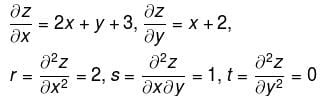For max. or min of z, we must have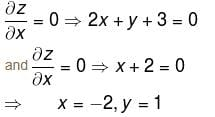at (–2, 1)
rt – s2 = –1 < 0
∴  neither max. nor min.
The correct answer is: neither max nor min

Differential Calculus MCQ Level - 1 - Question 2

###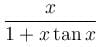is maximum at x= :

Detailed Solution for Differential Calculus MCQ Level - 1 - Question 2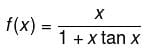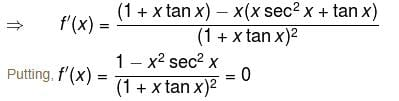We get, x = ±cos x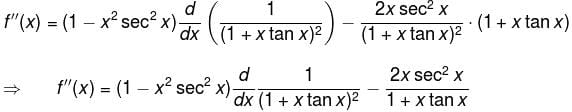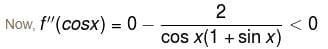∴ f(x) has maximum where x = cos x.

The correct answer is: cos x

Differential Calculus MCQ Level - 1 - Question 3

### The points on  z2 = xy + 1  nearest to the origin are :

Detailed Solution for Differential Calculus MCQ Level - 1 - Question 3

Let l be the distance from (0, 0, 0) to (x, y, z) on the surface
z2 = xy + 1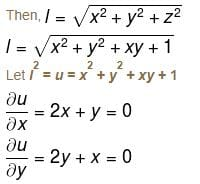Solving, we get
x = 0, y = 0
r = 2, s = 1, t = 2
rt – s2 = 3 > 0 and r > 0
Thus, minimum at (0, 0)
When, x = 0, y = 0, z = ±1
So, the points are (0, 0, 1) and (0, 0, –1)

The correct answer is: (0, 0, 1) and (0, 0, –1)

Differential Calculus MCQ Level - 1 - Question 4

The values of x1 and x2 with x1 < x2  such that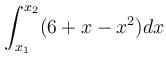has the largest value are :

Detailed Solution for Differential Calculus MCQ Level - 1 - Question 4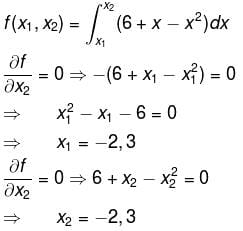Since, x1 < x2
So, x1 = –2  &  x2 = 3

The correct answer is: –2, 3

Differential Calculus MCQ Level - 1 - Question 5

The sum of two number is k. The minima value of the sum of their squares is :

Detailed Solution for Differential Calculus MCQ Level - 1 - Question 5

Let the two numbers be x and y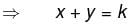and let z be the sum of squares.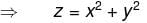x2 + (k – x)2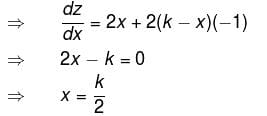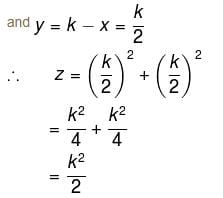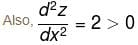Hence, z is minimum at x = k/2 and its minimum value is k2/2.

Differential Calculus MCQ Level - 1 - Question 6

The maximum value of f(x) = (1/x)x is

Detailed Solution for Differential Calculus MCQ Level - 1 - Question 6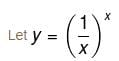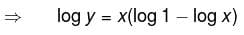= –x log x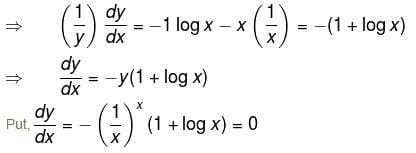i.e., 1 + log x = 0
i.e., log x = –1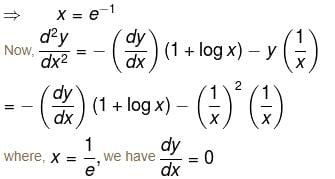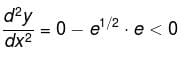Hence, y is maximum where x = 1/e and maximum value is e1/e.

Differential Calculus MCQ Level - 1 - Question 7

For the function x2 + y2,  (0, 0) is a point of :

Detailed Solution for Differential Calculus MCQ Level - 1 - Question 7

Let f(x, y) = x2 + y2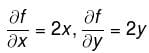For stationary points,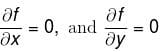⇒ (0, 0) is the only stationary point
r = fxx = 2, t = fyy = 2, s = fxy = 0
rt – s2 = 4 – 0 = 4 > 0 and r > 0
Thus, (0, 0) is a point of minima.

Differential Calculus MCQ Level - 1 - Question 8

If  dy/dx = (x - a)2n (x - b)2p + 1,  where n and p are positive integers, then at x = a, x = b, f(x) has respectively :

Detailed Solution for Differential Calculus MCQ Level - 1 - Question 8

For stationary points, put dy/dx = 0
i.e. (x – a)2n(x – b)2p+1 = 0
i.e. x = ab
Nature at x = a : Since 2n is even, dy/dx does not change its sign while x passes through a.

∴  Neither maximum nor minima at x = a.

Nature at x = b : when x is slightly less than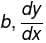is negative as 2p + 1 is odd.

Also, when x is slightly more than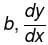is positive. Thus, y is minimum at x = b.

The correct answer is: neither maxima nor minima, minima

Differential Calculus MCQ Level - 1 - Question 9

The maximum value of (x – 1)(x – 2)(x – 3) is :

Detailed Solution for Differential Calculus MCQ Level - 1 - Question 9

f(y) = (x – 1)(x – 2)(x – 3)
= x3 – 6x2 + 11x – 6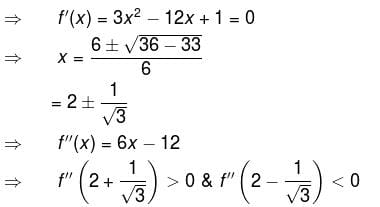Hence, f(x) has minima at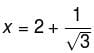and f(x) has maxima at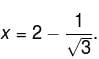∴  the maximum value of f(x) is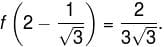The correct answer is: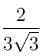Differential Calculus MCQ Level - 1 - Question 10

The saddle point of the function z = x2y – y2x – x + y is :

Detailed Solution for Differential Calculus MCQ Level - 1 - Question 10

For max. or min.,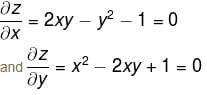Solving, we get
x = 1, y = 1
x = –1, y = –1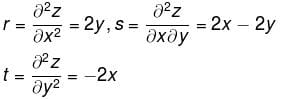at (1, 1)     r = 2, s = 0, t = –2
rt – s2 = –4 < 0

∴ neither max nor min at (1, 1)
at (–1, –1)  r = –2, s = 0, t = 2
rt – s2 = –4 < 0
Thus, (–1, –1) is also a saddle point.

The correct answer is: both (1, 1) and (–1, –1)

## Topic wise Tests for IIT JAM Physics

217 tests
Information about Differential Calculus MCQ Level - 1 Page
In this test you can find the Exam questions for Differential Calculus MCQ Level - 1 solved & explained in the simplest way possible. Besides giving Questions and answers for Differential Calculus MCQ Level - 1, EduRev gives you an ample number of Online tests for practice

## Topic wise Tests for IIT JAM Physics

217 tests(Scan QR code)